Ex.3.7 (Optional)

Chapter 3 Class 10 Pair of Linear Equations in Two Variables
Serial order wise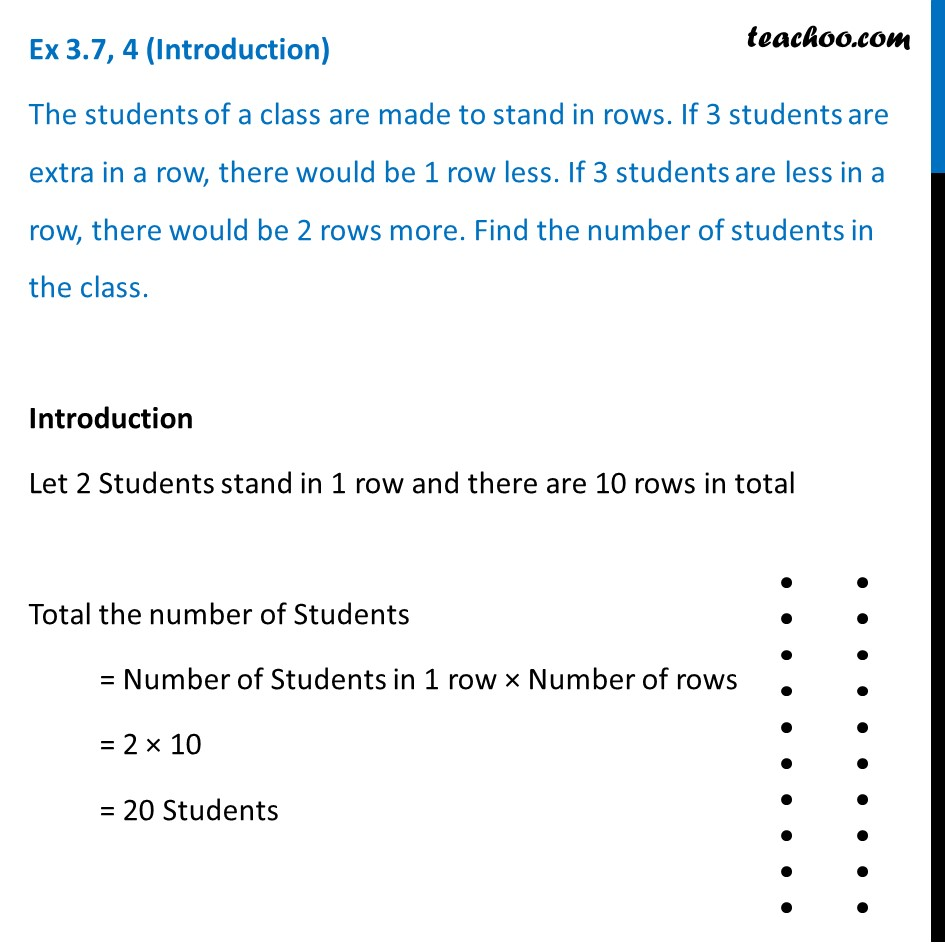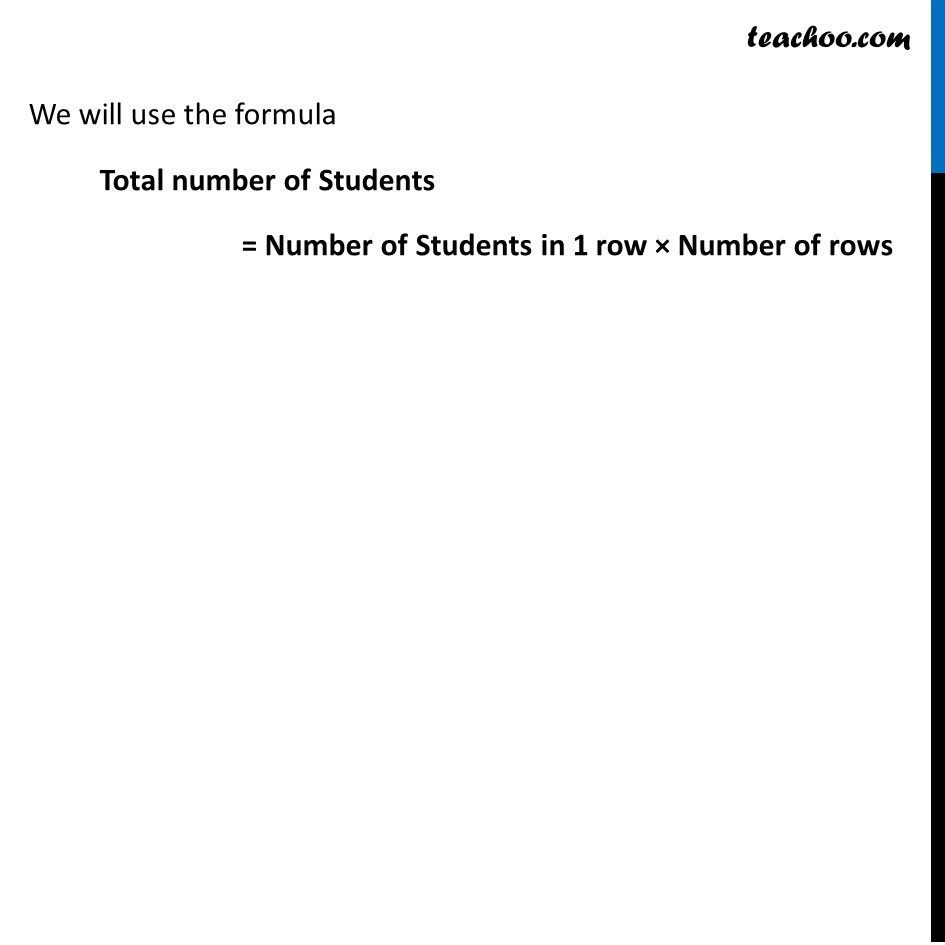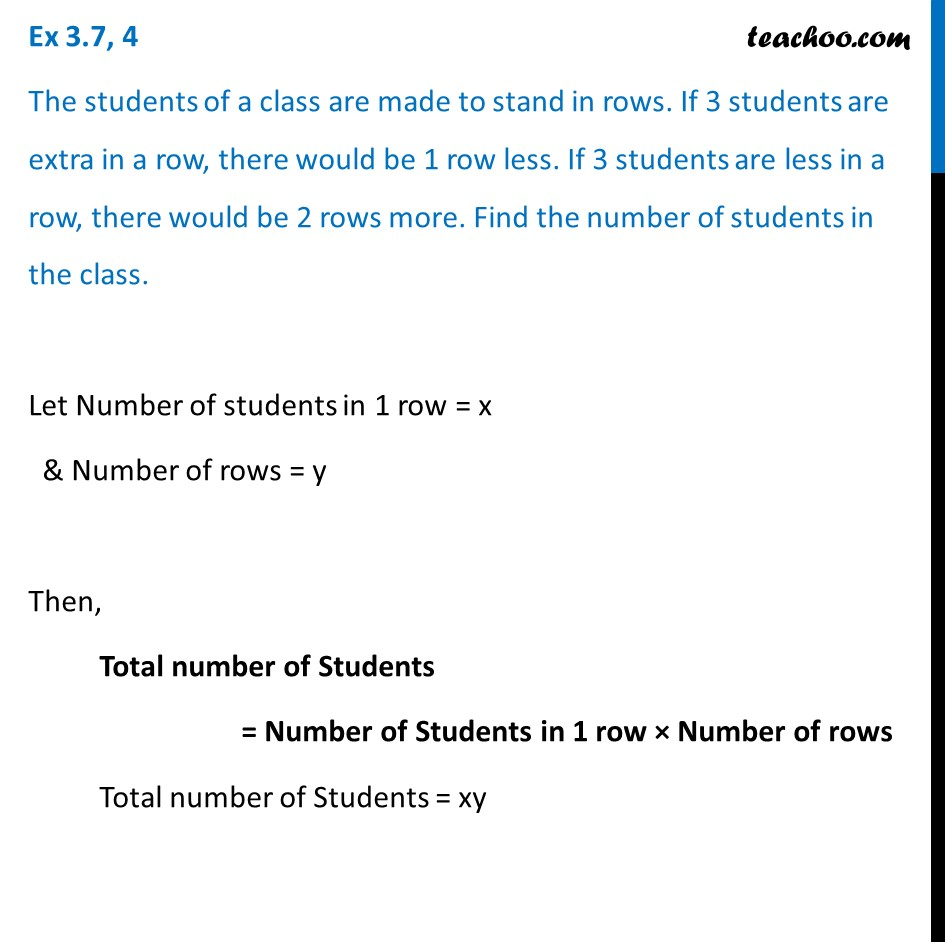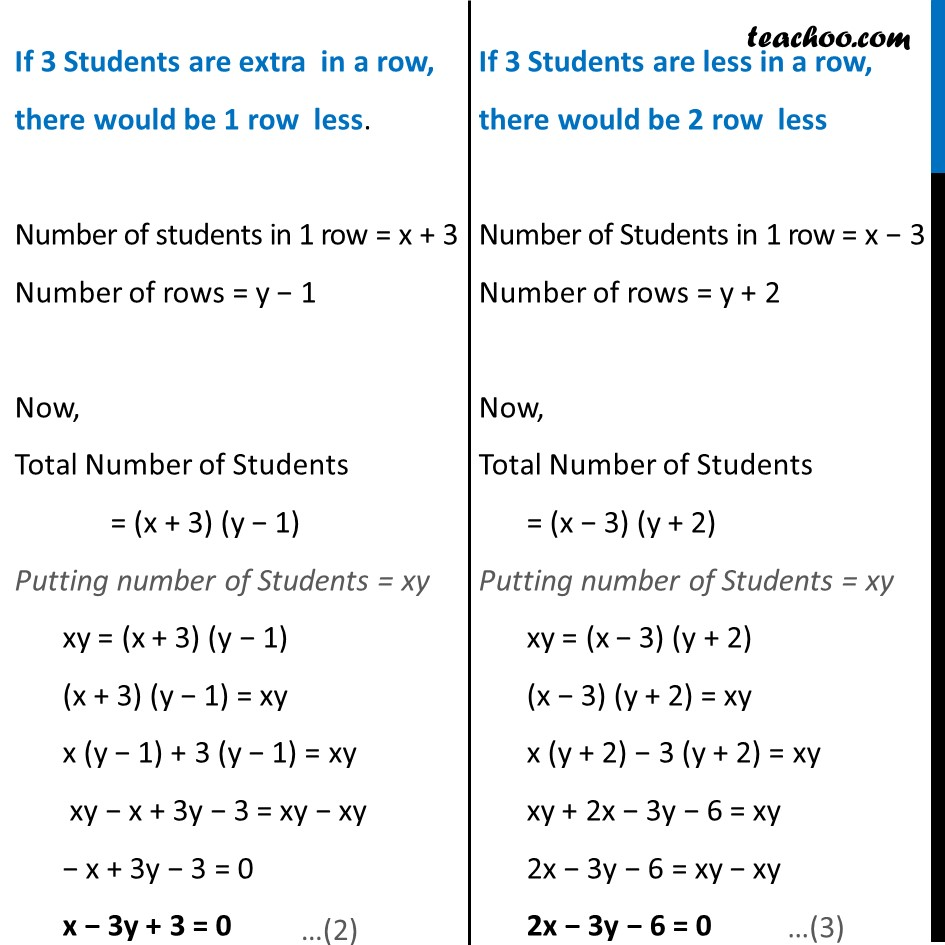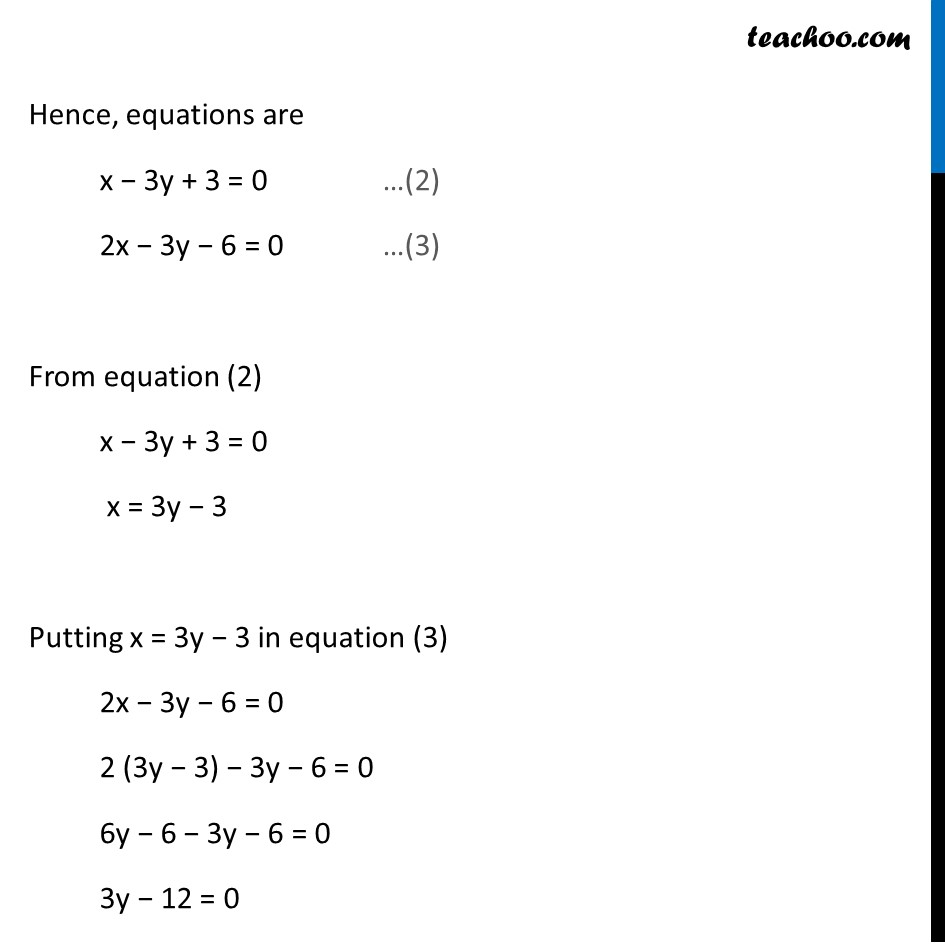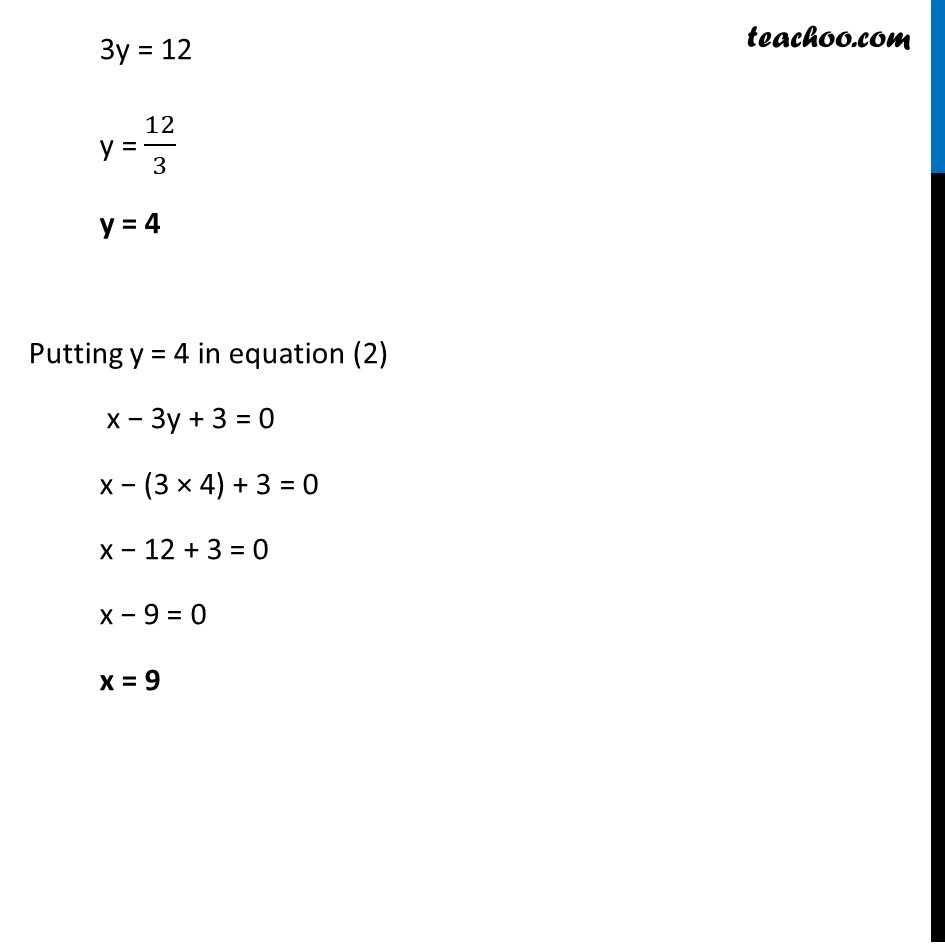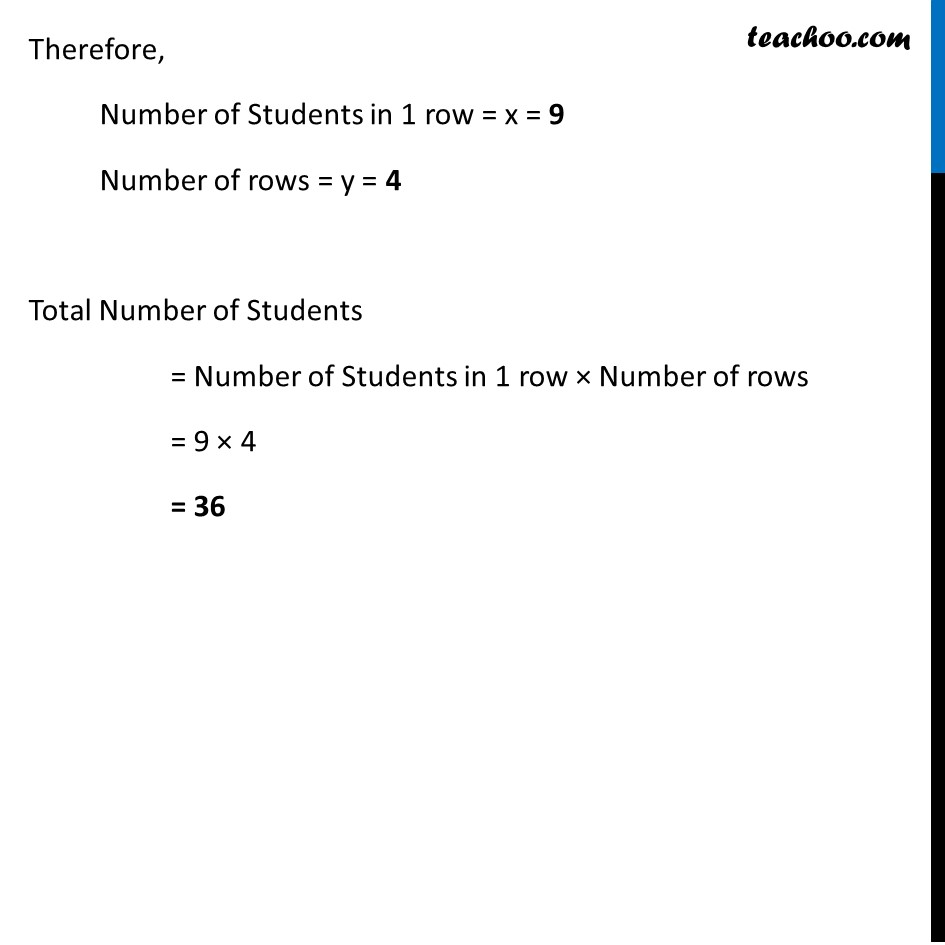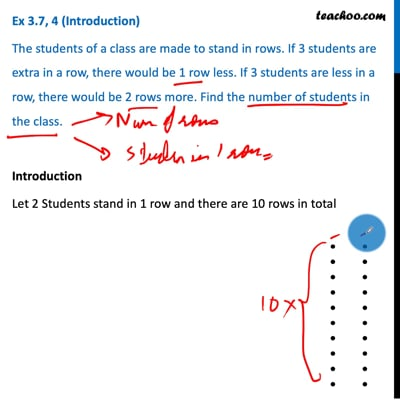This video is only available for Teachoo black users

Maths Crash Course - Live lectures + all videos + Real time Doubt solving!

### Transcript

Ex 3.7, 4 (Introduction) The students of a class are made to stand in rows. If 3 students are extra in a row, there would be 1 row less. If 3 students are less in a row, there would be 2 rows more. Find the number of students in the class. Introduction Let 2 Students stand in 1 row and there are 10 rows in total Total the number of Students = Number of Students in 1 row × Number of rows = 2 × 10 = 20 Students We will use the formula Total number of Students = Number of Students in 1 row × Number of rows Ex 3.7, 4 The students of a class are made to stand in rows. If 3 students are extra in a row, there would be 1 row less. If 3 students are less in a row, there would be 2 rows more. Find the number of students in the class. Let Number of students in 1 row = x & Number of rows = y Then, Total number of Students = Number of Students in 1 row × Number of rows Total number of Students = xy If 3 Students are extra in a row, there would be 1 row less. Number of students in 1 row = x + 3 Number of rows = y − 1 Now, Total Number of Students = (x + 3) (y − 1) Putting number of Students = xy xy = (x + 3) (y − 1) (x + 3) (y − 1) = xy x (y − 1) + 3 (y − 1) = xy xy − x + 3y − 3 = xy − xy − x + 3y − 3 = 0 x − 3y + 3 = 0 If 3 Students are less in a row, there would be 2 row less Number of Students in 1 row = x − 3 Number of rows = y + 2 Now, Total Number of Students = (x − 3) (y + 2) Putting number of Students = xy xy = (x − 3) (y + 2) (x − 3) (y + 2) = xy x (y + 2) − 3 (y + 2) = xy xy + 2x − 3y − 6 = xy 2x − 3y − 6 = xy − xy 2x − 3y − 6 = 0 Hence, equations are x − 3y + 3 = 0 …(2) 2x − 3y − 6 = 0 …(3) From equation (2) x − 3y + 3 = 0 x = 3y − 3 Putting x = 3y − 3 in equation (3) 2x − 3y − 6 = 0 2 (3y − 3) − 3y − 6 = 0 6y − 6 − 3y − 6 = 0 3y − 12 = 0 3y = 12 y = 12/3 y = 4 Putting y = 4 in equation (2) x − 3y + 3 = 0 x − (3 × 4) + 3 = 0 x − 12 + 3 = 0 x − 9 = 0 x = 9 Therefore, Number of Students in 1 row = x = 9 Number of rows = y = 4 Total Number of Students = Number of Students in 1 row × Number of rows = 9 × 4 = 36